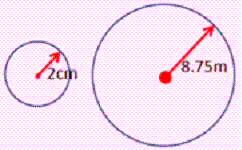Name:    Math 9 7.1 to 7.3 Online Quiz

True/False
Indicate whether the statement is true or false.

1.

TRUE/FALSE:  If two triangles are similar, they have the same shape.

2.

TRUE/FALSE:  If two triangles are similar, they have the same size

3.

TRUE/FALSE: All equilateral triangles are similar

4.

TRUE/FALSE All isosceles right triangles are similar

5.

TRUE/FALSE All right triangles are similar

Multiple Choice
Identify the choice that best completes the statement or answers the question.

6.

Given each of the following equations, find the value of “x”: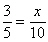a. 3 c. 6 b. 4 d. 8

7.

Given each of the following equations, find the value of “x”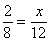a. 2 c. 4 b. 3 d. 6

8.

Given each of the following equations, find the value of “x”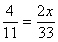a. 12 c. 6 b. 8 d. 3

9.

What is the scale factor for the following Eiffel tower model?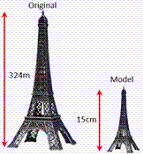a. 0.0463 c. 0.000463 b. 21.6 d. 2,160

10.

The average length of a BMW 325xi is about 4.85meters long.  A toy model of this car is reduced at a scaled factor of 0.25.  What is the length of the toy model?
 a. 19.4m c. 1.25m b. 1.2125m d. 18.75m

11.

The distance between Vancouver and Seattle is 226.5km.  The distance between the two cities on a map is about 2.3cm apart.  What is the scaled factor of the map?
 a. 0.010154 c. 985,000 b. 98.5 d. 0.000,000,10154

12.

An architect builds a model of a building at a scaled factor of 0.0003.  If the model has a length of 85cm long and 75cm wide, what is the length and width of the actual building in meters? What is the area of the building?
 a.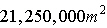c.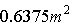b.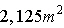d.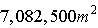13.

Given that the following polygons are similar, find the length of PT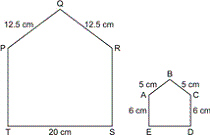a.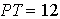cm c.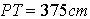b.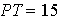cm d.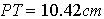14.

Find the length of RS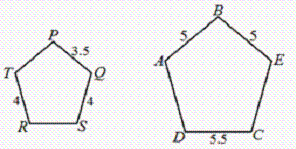a.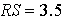c.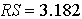b.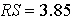d.Yes/No
Indicate whether you agree with the statement.

15.

Are the following shapes similar?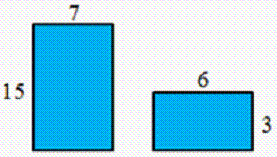16.

Are the following shapes similar?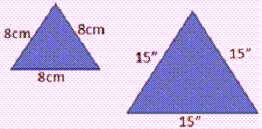17.

Are the following shapes similar?